# NCERT Exemplar Class 12 Physics Solutions for Chapter 13 Nuclei

NCERT Class 12 Physics Exemplar Solutions for Chapter 13 Nuclei are prepared by the highly experienced faculty at BYJU’S. The NCERT Class 12 Physics Exemplar Solutions for Chapter 13 aid in preparing for the board examination, as the question papers of the CBSE board are set according to the prescribed syllabus. Solving these exemplar questions and problems helps students clear all their doubts on the chapter Nuclei.

This exemplar page has answers to questions in the exemplar book, along with extra questions prepared by our subject experts, questions from previous years’ question papers, sample papers and other resources. This exemplar solution helps you to understand the concepts and score well in the board exam.

## Download the PDF of the NCERT Exemplar for Class 12 Physics Chapter 13 Nuclei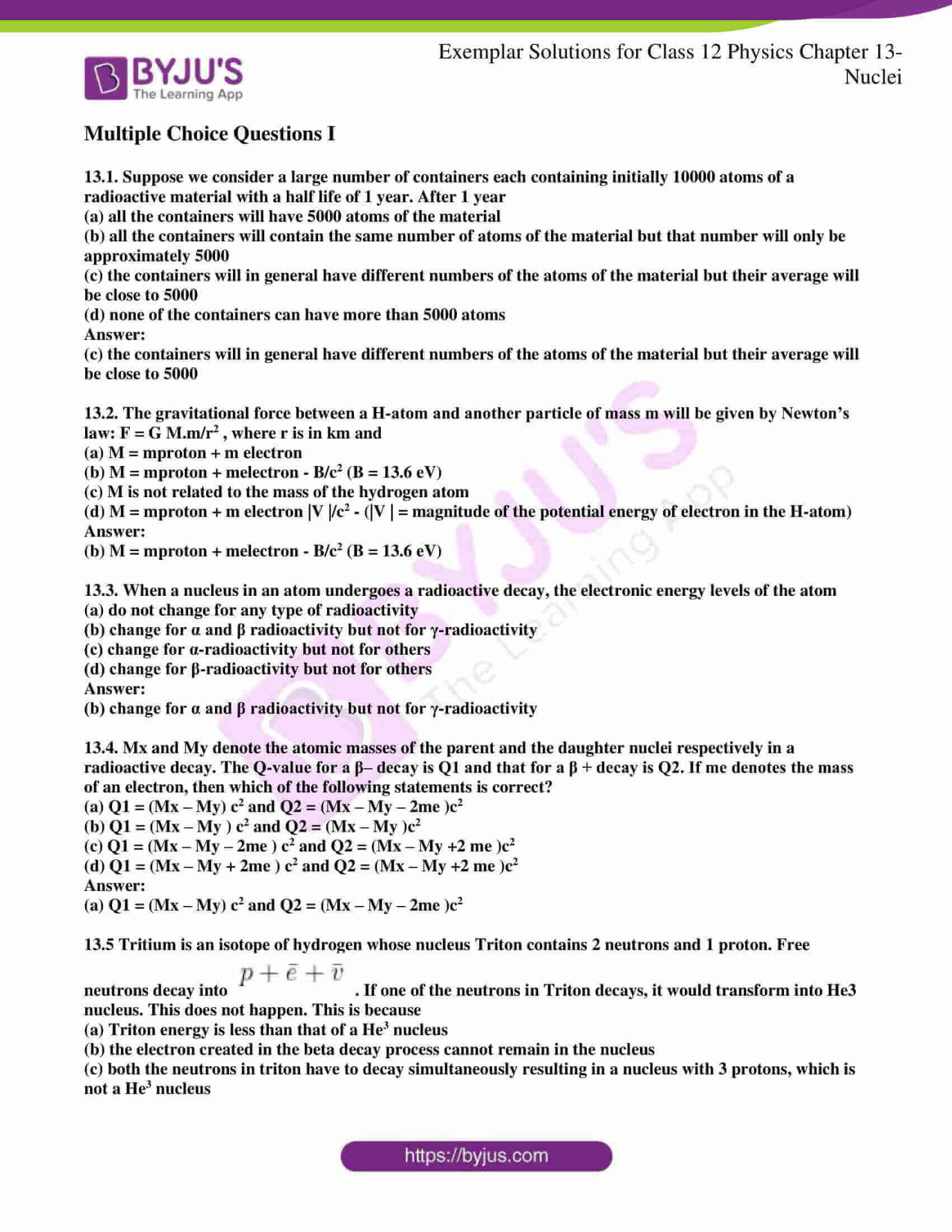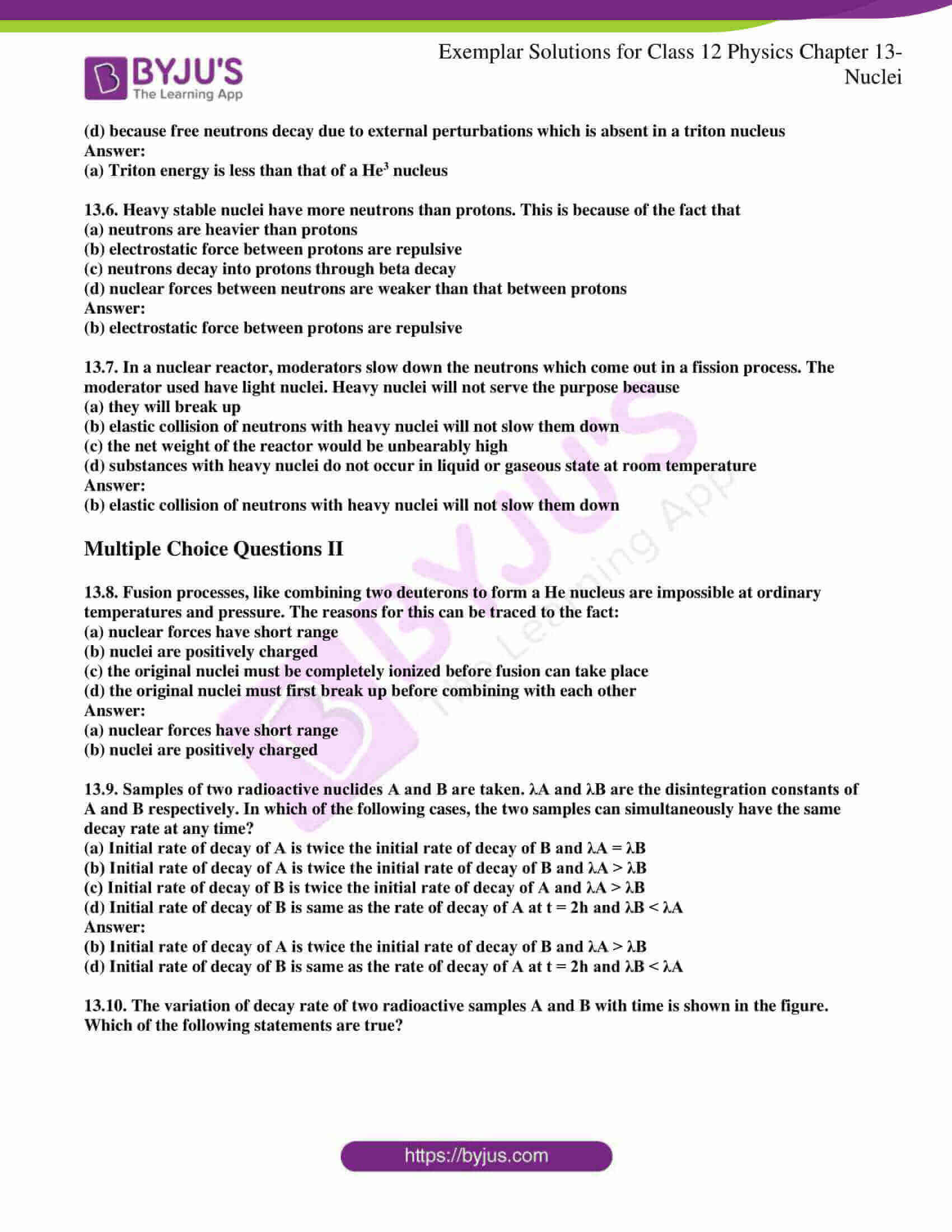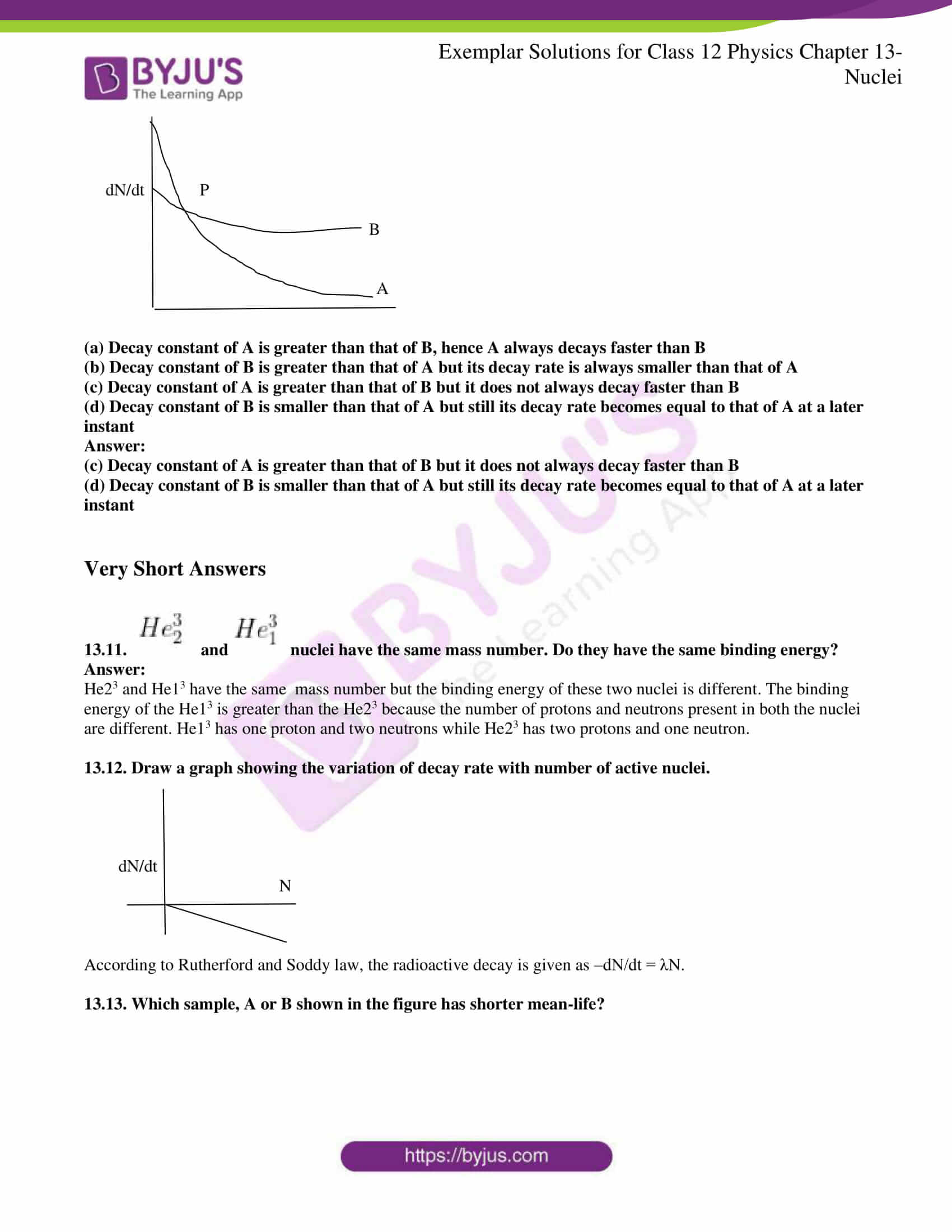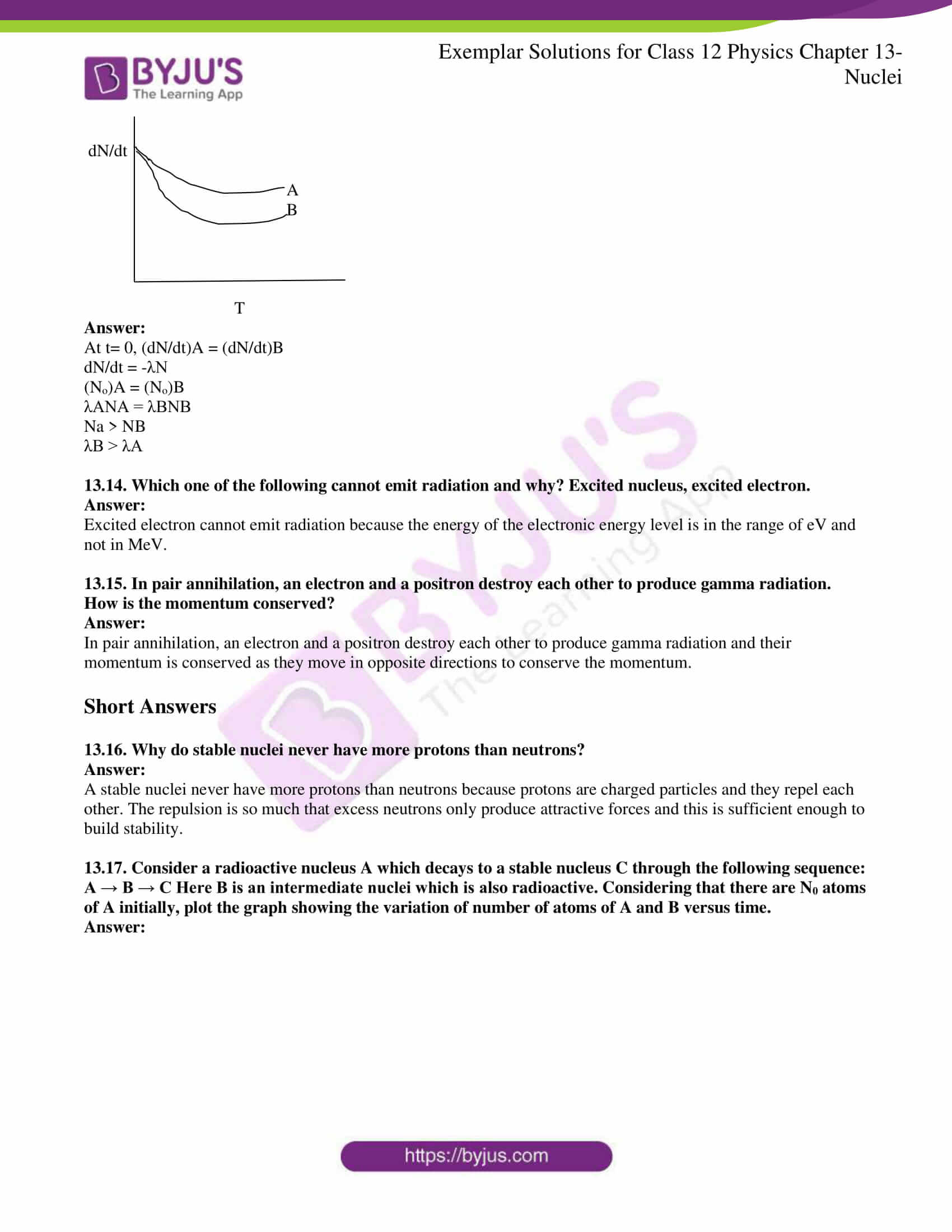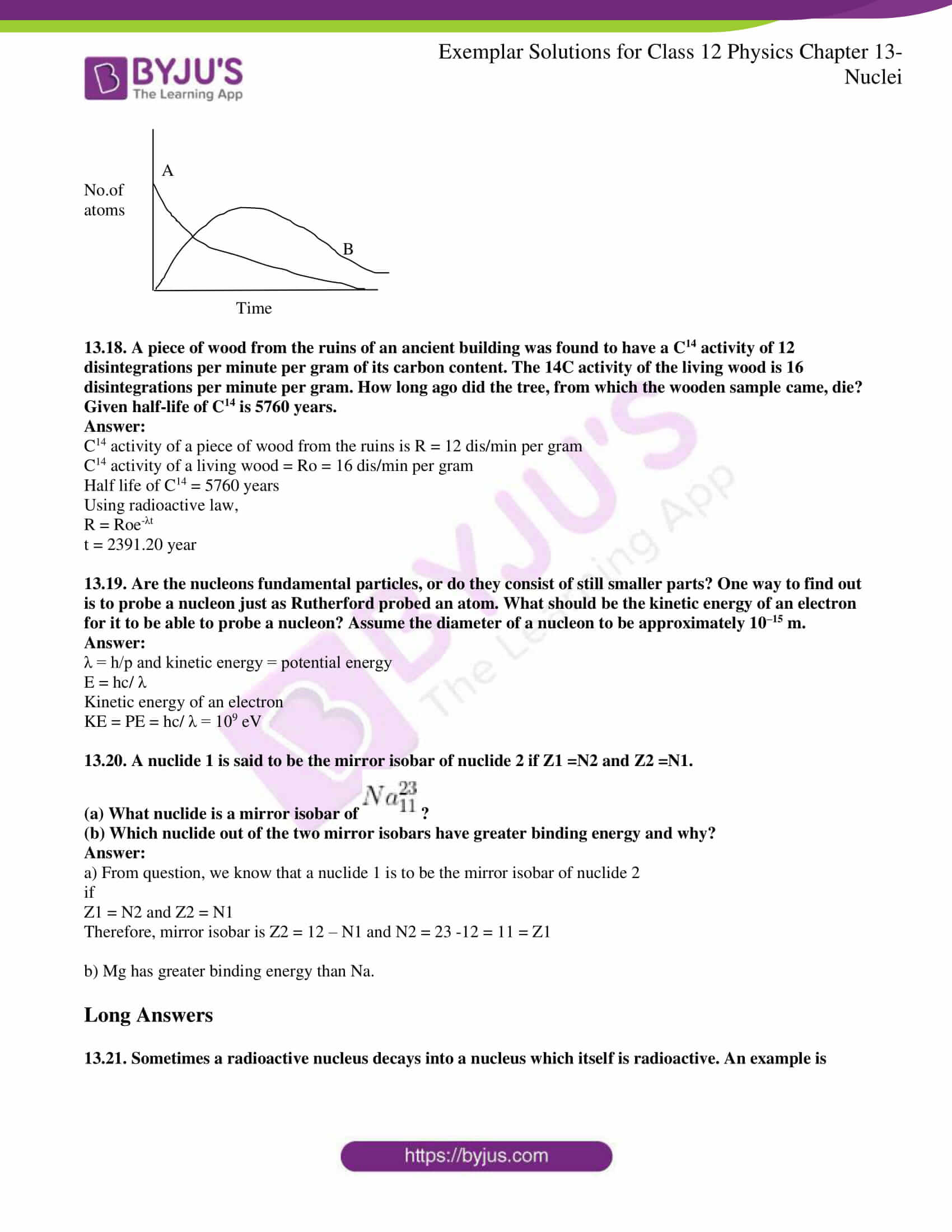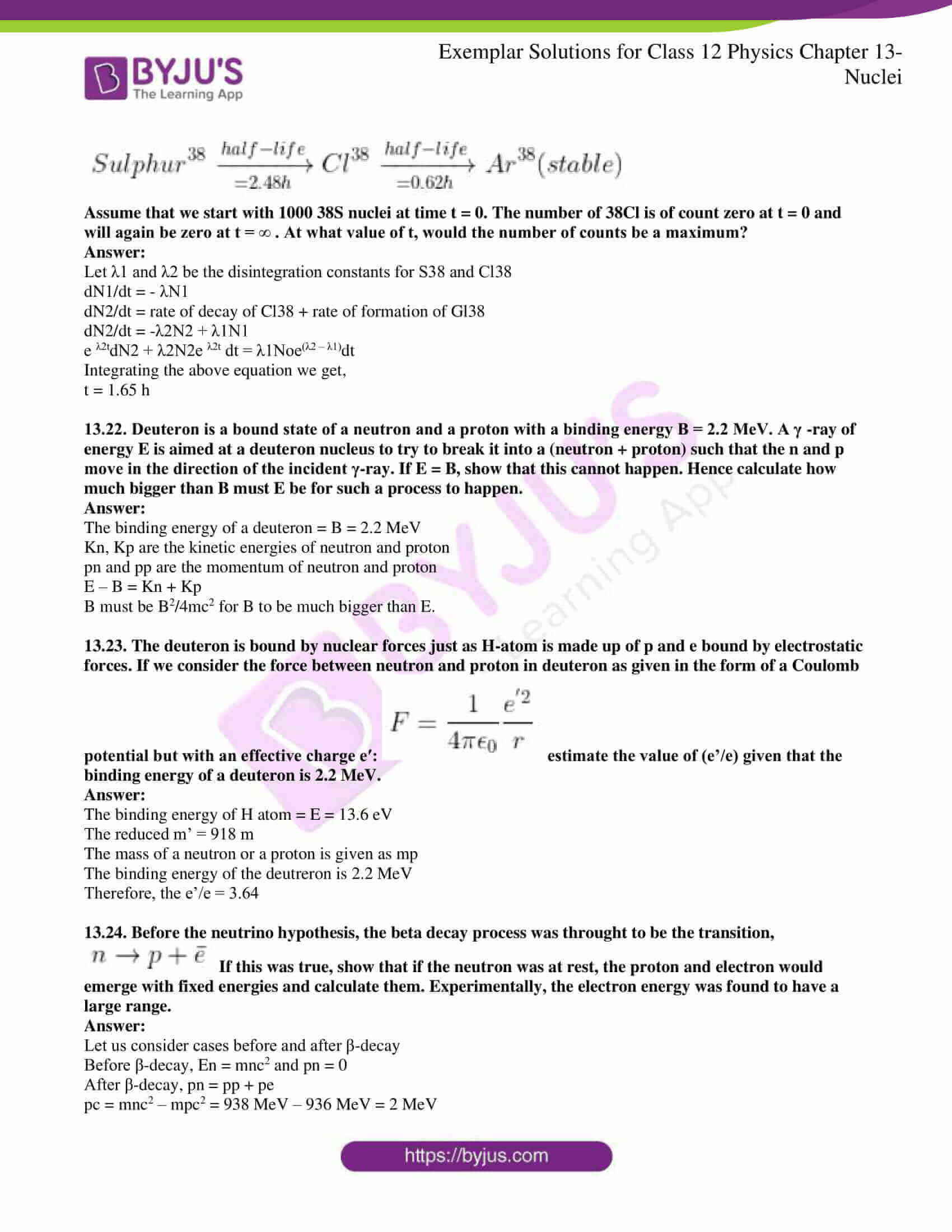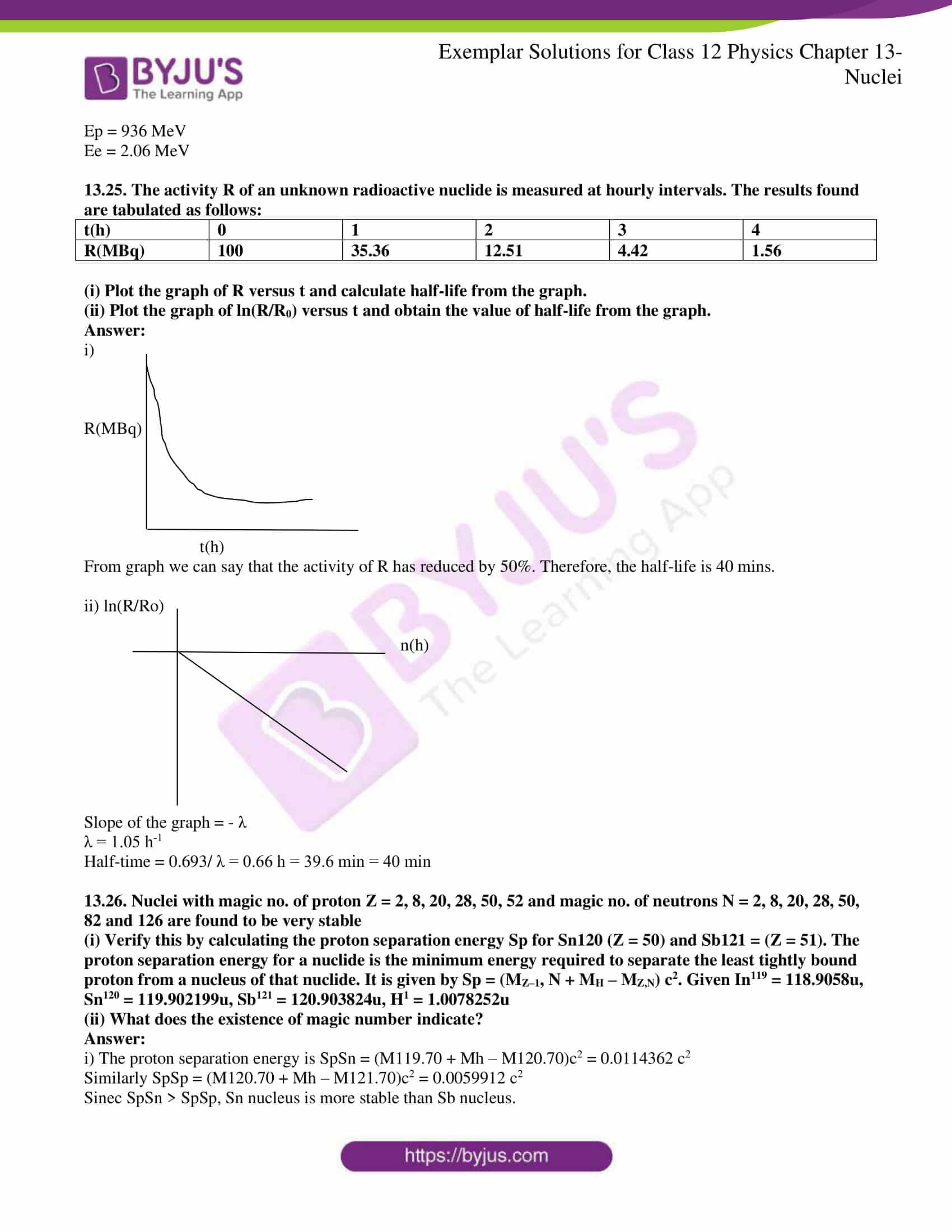### Multiple Choice Questions I

13.1. Suppose we consider a large number of containers each containing initially 10000 atoms of a radioactive material with a half-life of 1 year. After 1 year

(a) all the containers will have 5000 atoms of the material

(b) all the containers will contain the same number of atoms of the material but that number will only be approximately 5000

(c) the containers will, in general, have different numbers of the atoms of the material but their average will be close to 5000

(d) none of the containers can have more than 5000 atoms

(c) the containers will, in general, have different numbers of the atoms of the material but their average will be close to 5000

13.2. The gravitational force between an H-atom and another particle of mass m will be given by Newton’s law: F = G M.m/r2 , where r is in km and

(a) M = mproton + m electron

(b) M = mproton + melectron – B/c2 (B = 13.6 eV)

(c) M is not related to the mass of the hydrogen atom

(d) M = mproton + m electron |V |/c2 – (|V | = magnitude of the potential energy of electron in the H-atom)

(b) M = mproton + melectron – B/c2 (B = 13.6 eV)

13.3. When a nucleus in an atom undergoes radioactive decay, the electronic energy levels of the atom

(a) do not change for any type of radioactivity

(c) change for α-radioactivity but not for others

(d) change for β-radioactivity but not for others

13.4. Mx and My denote the atomic masses of the parent and the daughter nuclei respectively in a radioactive decay. The Q-value for a β– decay is Q1 and that for a β + decay is Q2. If me denotes the mass of an electron, then which of the following statements is correct?

(a) Q1 = (Mx – My) c2 and Q2 = (Mx – My – 2me )c2

(b) Q1 = (Mx – My ) c2 and Q2 = (Mx – My )c2

(c) Q1 = (Mx – My – 2me ) c2 and Q2 = (Mx – My +2 me )c2

(d) Q1 = (Mx – My + 2me ) c2 and Q2 = (Mx – My +2 me )c2

(a) Q1 = (Mx – My) c2 and Q2 = (Mx – My – 2me )c2

13.5 Tritium is an isotope of hydrogen whose nucleus Triton contains 2 neutrons and 1 proton. Free neutrons decay into

$$\begin{array}{l}P +\bar{e}+\bar{\upsilon}\end{array}$$
. If one of the neutrons in Triton decays, it would transform into He3 nucleus. This does not happen. This is because

(a) Triton energy is less than that of a He3 nucleus

(b) the electron created in the beta decay process cannot remain in the nucleus

(c) both the neutrons in triton have to decay simultaneously resulting in a nucleus with 3 protons, which is not a He3 nucleus

(d) because free neutrons decay due to external perturbations which is absent in a triton nucleus

(a) Triton energy is less than that of a He3 nucleus

13.6. Heavy stable nuclei have more neutrons than protons. This is because of the fact that

(a) neutrons are heavier than protons

(b) electrostatic force between protons are repulsive

(c) neutrons decay into protons through beta decay

(d) nuclear forces between neutrons are weaker than that between protons

(b) electrostatic force between protons are repulsive

13.7. In a nuclear reactor, moderators slow down the neutrons which come out in a fission process. The moderator used to have light nuclei. Heavy nuclei will not serve the purpose because

(a) they will break up

(b) elastic collision of neutrons with heavy nuclei will not slow them down

(c) the net weight of the reactor would be unbearably high

(d) substances with heavy nuclei do not occur in a liquid or gaseous state at room temperature

(b) elastic collision of neutrons with heavy nuclei will not slow them down

### Multiple Choice Questions II

13.8. Fusion processes, like combining two deuterons to form a He nucleus are impossible at ordinary temperatures and pressure. The reasons for this can be traced to the fact:

(a) nuclear forces have short-range

(b) nuclei are positively charged

(c) the original nuclei must be completely ionized before fusion can take place

(d) the original nuclei must first break up before combining with each other

(a) nuclear forces have short-range

(b) nuclei are positively charged

13.9. Samples of two radioactive nuclides A and B are taken. λA and λB are the disintegration constants of A and B respectively. In which of the following cases, the two samples can simultaneously have the same decay rate at any time?

(a) Initial rate of decay of A is twice the initial rate of decay of B and λA = λB

(b) Initial rate of decay of A is twice the initial rate of decay of B and λA > λB

(c) Initial rate of decay of B is twice the initial rate of decay of A and λA > λB

(d) Initial rate of decay of B is the same as the rate of decay of A at t = 2h and λB < λA

(b) Initial rate of decay of A is twice the initial rate of decay of B and λA > λB

(d) Initial rate of decay of B is the same as the rate of decay of A at t = 2h and λB < λA

13.10. The variation of the decay rate of two radioactive samples A and B with time is shown in the figure. Which of the following statements are true?

dN/dt P

B

A

(a) The decay constant of A is greater than that of B, hence A always decays faster than B

(b) The decay constant of B is greater than that of A but its decay rate is always smaller than that of A

(c) The decay constant of A is greater than that of B but it does not always decay faster than B

(d) The decay constant of B is smaller than that of A but still, its decay rate becomes equal to that of A at a later instant

(c) The decay constant of A is greater than that of B but it does not always decay faster than B

(d) The decay constant of B is smaller than that of A but still, its decay rate becomes equal to that of A at a later instant

13.11.

$$\begin{array}{l}He_{2}^{3}\end{array}$$
and
$$\begin{array}{l}He_{1}^{3}\end{array}$$
nuclei have the same mass number. Do they have the same binding energy?

He23 and He13 have the same mass number but the binding energy of these two nuclei is different. The binding energy of the He13 is greater than the He23 because the number of protons and neutrons present in both the nuclei are different. He13 has one proton and two neutrons while He23 has two protons and one neutron.

13.12. Draw a graph showing the variation of decay rate with number of active nuclei.

dN/dt

N

According to Rutherford and Soddy law, the radioactive decay is given as –dN/dt = λN.

13.13. Which sample, A or B shown in the figure has shorter mean-life?

dN/dt

A

B

T

At t= 0, (dN/dt)A = (dN/dt)B

dN/dt = -λN

(No)A = (No)B

λANA = λBNB

Na > NB

λB > λA

13.14. Which one of the following cannot emit radiation and why? Excited nucleus, excited electron.

An excited electron cannot emit radiation because the energy of the electronic energy level is in the range of eV and not in MeV.

13.15. In pair annihilation, an electron and a positron destroy each other to produce gamma radiation. How is the momentum conserved?

In pair annihilation, an electron and a positron destroy each other to produce gamma radiation and their momentum is conserved as they move in opposite directions to conserve the momentum.

13.16. Why do stable nuclei never have more protons than neutrons?

A stable nuclei never have more protons than neutrons because protons are charged particles and they repel each other. The repulsion is so much that excess neutrons only produce attractive forces and this is sufficient enough to build stability.

13.17. Consider a radioactive nucleus A which decays to a stable nucleus C through the following sequence: A → B → C Here B is an intermediate nuclei which is also radioactive. Considering that there are N0 atoms of A initially, plot the graph showing the variation of a number of atoms of A and B versus time.

A

No.of

atoms

B

Time

13.18. A piece of wood from the ruins of an ancient building was found to have a C14 activity of 12 disintegrations per minute per gram of its carbon content. The 14C activity of the living wood is 16 disintegrations per minute per gram. How long ago did the tree, from which the wooden sample came, die? Given the half-life of C14 is 5760 years.

C14 activity of a piece of wood from the ruins is R = 12 dis/min per gram

C14 activity of a living wood = Ro = 16 dis/min per gram

Half-life of C14 = 5760 years

R = Roe-λt

t = 2391.20 year

13.19. Are the nucleons fundamental particles, or do they consist of still smaller parts? One way to find out is to probe a nucleon just as Rutherford probed an atom. What should be the kinetic energy of an electron for it to be able to probe a nucleon? Assume the diameter of a nucleon to be approximately 10–15 m.

λ = h/p and kinetic energy = potential energy

E = hc/ λ

Kinetic energy of an electron

KE = PE = hc/ λ = 109 eV

13.20. A nuclide 1 is said to be the mirror isobar of nuclide 2 if Z1 =N2 and Z2 =N1.

(a) What nuclide is a mirror isobar of

$$\begin{array}{l}Na_{11}^{23}\end{array}$$
?

(b) Which nuclide out of the two mirror isobars have greater binding energy and why?

a) From the question, we know that a nuclide 1 is to be the mirror isobar of nuclide 2

if

Z1 = N2 and Z2 = N1

Therefore, mirror isobar is Z2 = 12 – N1 and N2 = 23 -12 = 11 = Z1

b) Mg has greater binding energy than Na.

13.21. Sometimes a radioactive nucleus decays into a nucleus which itself is radioactive. An example is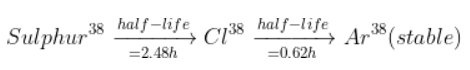Assume that we start with 1000 38S nuclei at time t = 0. The number of 38Cl is of count zero at t = 0 and will again be zero at t = ∞ . At what value of t, would the number of counts be a maximum?

Let λ1 and λ2 be the disintegration constants for S38 and Cl38

dN1/dt = – λN1

dN2/dt = rate of decay of Cl38 + rate of formation of Gl38

dN2/dt = -λ2N2 + λ1N1

e λ2tdN2 + λ2N2e λ2t dt = λ1Noe(λ2 – λ1)dt

Integrating the above equation we get,

t = 1.65 h

13.22. Deuteron is a bound state of a neutron and a proton with a binding energy B = 2.2 MeV. A γ -ray of energy E is aimed at a deuteron nucleus to try to break it into a (neutron + proton) such that the n and p move in the direction of the incident γ-ray. If E = B, show that this cannot happen. Hence calculate how much bigger than B must E be for such a process to happen.

The binding energy of a deuteron = B = 2.2 MeV

Kn, Kp are the kinetic energies of neutron and proton

pn and pp are the momentum of neutron and proton

E – B = Kn + Kp

B must be B2/4mc2 for B to be much bigger than E.

13.23. The deuteron is bound by nuclear forces just as H-atom is made up of p and e bound by electrostatic forces. If we consider the force between neutron and proton in deuteron as given in the form of a Coulomb potential but with an effective charge e′:

$$\begin{array}{l}F=\frac{1}{4\Pi \epsilon_{0}}\frac{e’^{2}}{r}\end{array}$$
estimate the value of (e’/e) given that the binding energy of a deuteron is 2.2 MeV.

The binding energy of H atom = E = 13.6 eV

The reduced m’ = 918 m

The mass of a neutron or a proton is given as mp

The binding energy of the deuteron is 2.2 MeV

Therefore, the e’/e = 3.64

13.24. Before the neutrino hypothesis, the beta decay process was thought to be the transition,

$$\begin{array}{l}n\rightarrow P+\bar{e}\end{array}$$
If this was true, show that if the neutron was at rest, the proton and electron would emerge with fixed energies and calculate them. Experimentally, the electron energy was found to have a large range.

Let us consider cases before and after β-decay

Before β-decay, En = mnc2 and pn = 0

After β-decay, pn = pp + pe

pc = mnc2 – mpc2 = 938 MeV – 936 MeV = 2 MeV

Ep = 936 MeV

Ee = 2.06 MeV

13.25. The activity R of an unknown radioactive nuclide is measured at hourly intervals. The results found are tabulated as follows:

 t(h) 0 1 2 3 4 R(MBq) 100 35.36 12.51 4.42 1.56

(i) Plot the graph of R versus t and calculate half-life from the graph.

(ii) Plot the graph of ln(R/R0) versus t and obtain the value of half-life from the graph.

i)

R(MBq)

t(h)

From graph we can say that the activity of R has reduced by 50%. Therefore, the half-life is 40 mins.

ii) ln(R/Ro)

n(h)

Slope of the graph = – λ

λ = 1.05 h-1

Half-time = 0.693/ λ = 0.66 h = 39.6 min = 40 min

13.26. Nuclei with magic no. of proton Z = 2, 8, 20, 28, 50, 52 and magic no. of neutrons N = 2, 8, 20, 28, 50, 82 and 126 are found to be very stable

(i) Verify this by calculating the proton separation energy Sp for Sn120 (Z = 50) and Sb121 = (Z = 51). The proton separation energy for a nuclide is the minimum energy required to separate the least tightly bound proton from a nucleus of that nuclide. It is given by Sp = (MZ–1, N + MH – MZ,N) c2. Given In119 = 118.9058u, Sn120 = 119.902199u, Sb121 = 120.903824u, H1 = 1.0078252u

(ii) What does the existence of magic number indicate?

i) The proton separation energy is SpSn = (M119.70 + Mh – M120.70)c2 = 0.0114362 c2

Similarly SpSp = (M120.70 + Mh – M121.70)c2 = 0.0059912 c2

Since SpSn > SpSp, Sn nucleus is more stable than Sb nucleus.

ii) The magic numbers indicate that the shell structure of the nucleus is similar to the shell structure of the atom. This explains the peaks in the binding energy.

The central core present inside an atom is called the nucleus, while electrons are negatively charged particles that surround it. The incredibly strong force that keeps the nucleus together is called nuclear energy. The nucleus has a neutral charge, unlike the protons, which exhibit a positive charge. The mass of the proton is quite similar to that of a nucleus.

#### Concepts Discussed in Class 12 Chapter 13 Nuclei

1. Introduction
2. Atomic Masses and Composition of Nucleus
3. Size of the Nucleus
4. Mass-energy and Nuclear Binding Energy
1. Mass-energy
2. Nuclear binding energy
5. Nuclear Force
2. Alpha decay
3. Beta-decay
4. Gamma decay
7. Nuclear Energy
1. Fission
2. Nuclear reactor
3. Nuclear fusion – Energy generation in stars
4. Controlled thermonuclear fusion

Check out the NCERT Exemplar Class 12 Physics Chapter 13 Nuclei, available in PDF format below.

### Why Opt for BYJU’S

BYJU’S provides Class 12 Physics important questions, Class 12 Physics video and animation lessons, lecture notes, assignments and exercises to help students with their Class 12 and entrance exams preparation. They can also benefit from the study resources, as the study materials help them in scoring good marks in the board examination.